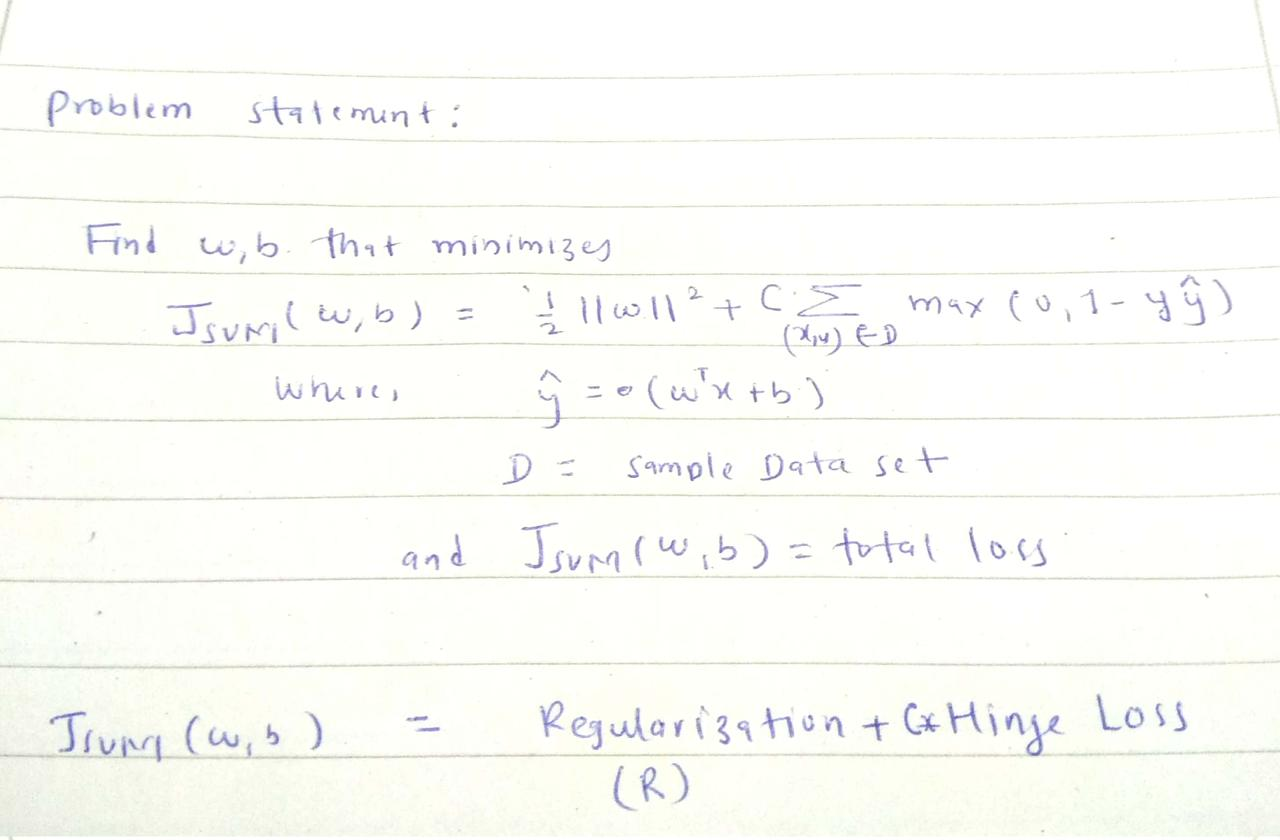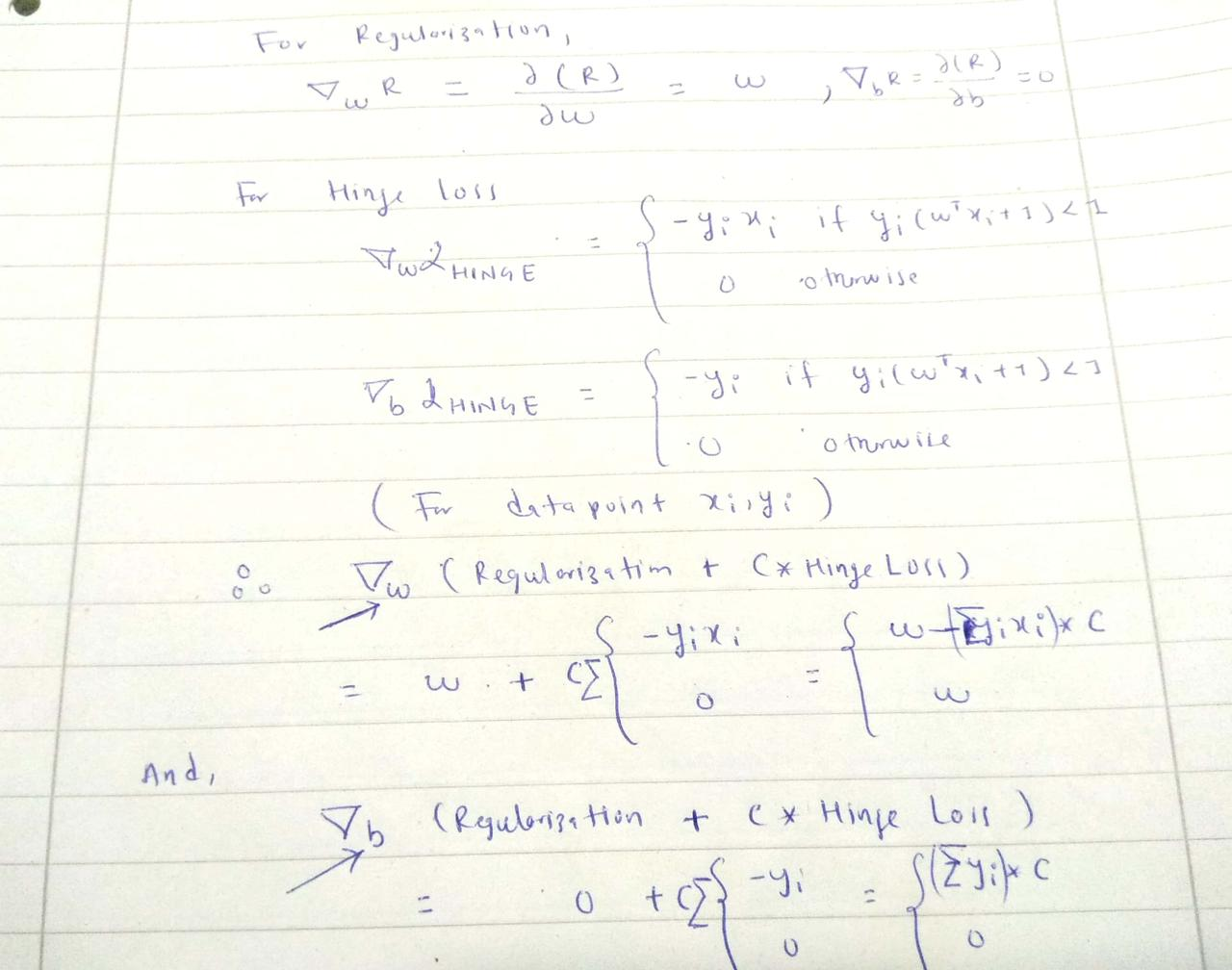In this post, we will see how we can train support vector machines using stochastic gradient descent (SGD). Before jumping to the algorithm, we need to know why subgradients?

Here is a brief summary of the 0-1 loss and hinge loss:But, why don't we use 0-1 loss ?? The obvious reason is it is not convex. Another factor could be it's reaction to small changes in parameters. You can see from the graph, if you change (w,b) the loss will flip to either 0 or 1 very fast without acknowledging the in between values. The hinge loss,on the other hand, has smooth change until it reaches '1' along x-axis.In :
import pandas as pd
import numpy as np

In :
def add_regularization(w, subgradient_w):
"""
The total loss :( 1/2 * ||w||^2 + Hingle_loss) has w term to be added after getting subgradient of 'w'

i.e total_w = w + C *  ∑ (-y*x)

"""

In :
def subgradients(x, y, w, b, C):
"""
:x: inputs [[x1,x2], [x2,x2],...]
:y: labels [1, -1,...]
:w: initial w
:b: initial b

"""

# sum over all subgradients of hinge loss for a given samples x,y
for x_i, y_i in zip(x,y):
f_xi = np.dot(w.T, x_i) + b

decision_value = y_i * f_xi

if decision_value < 1:
else:

# multiply by C after summation of all subgradients for a given samples of x,y

In :
def stochastic_subgrad_descent(data, initial_values, B, C, T=1):
"""
:data: Pandas data frame
:initial_values: initialization for w and b
:B: sample size for random data selection
:C: hyperparameter, tradeoff between hard margin and hinge loss
:T: # of iterations

"""
w, b = initial_values
for t in range(1, T+1):

# randomly select B data points
training_sample = data.sample(B)

# set learning rate
learning_rate = 1/t

# prepare inputs in the form [[h1, w1], [h2, w2], ....]
x = training_sample[['height', 'weight']].values

# prepare labels in the form [1, -1, 1, 1, - 1 ......]
y = training_sample['gender'].values

# update weights
w = w - learning_rate * sub_grads

# update bias
b = b - learning_rate * sub_grads
return (w,b)

In :
data = pd.DataFrame()

data['height'] = np.random.randint(160, 190, 100)
data['weight'] = np.random.randint(50, 90, 100)

data['gender'] = [-1 if value < 5 else 1 for value in np.random.randint(0,10, 100)]


Out:
height weight gender
0 167 82 1
1 183 57 -1
2 184 50 -1
3 178 53 -1
4 166 72 1
In :
initial_weights = np.array([-2, -3])
initial_bias = 12

initial_values = (initial_weights, initial_bias)

In :
w,b = stochastic_subgrad_descent(data, initial_values, 20, 1, 1000)

In :
w,b

Out:
(array([-0.798,  4.648]), 14.891692339980313)
In [ ]: